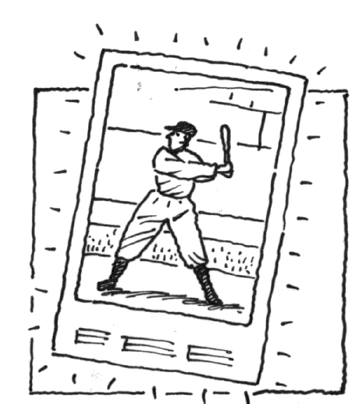### Home > APCALC > Chapter 6 > Lesson 6.4.2 > Problem6-133

6-133.

WHAT A CARD!

The value, $V$, of a Bonus Ragnew baseball card in mint condition appreciates at $12\%$ per year. It was sold at auction in 2001 for $461,000$. If $t =$ time in years since 2001, then $V = 461000(1.12)^t$.

1. What was its value in 2010?

We are given a value function, $V(t)$. Notice that $t =$ years since 1991. Evaluate for the year 2000.

2. Calculate the average value of the card over the period 2001 – 2010.

$\text{Value} = V(t) = 461,100(1.12)^t$

Examine the equation above:

What is the annual interest rate?

What is the initial value of the card?

$\text{Average value}=\frac{\Delta \text{value}}{\Delta \text{time}}$

3. When was the card worth the value you found in part (b)?

Unlike average value (AROC), actual value (IROC) must be found with a derivative.

Let $V^\prime(t) =$ your answer in part (b).

The card was worth its average value in 1995 or 1996.